Question

A 3.1 g sample of sodium hydrogen carbonate is added to a solution of acetic acid weighing 10.5 g. The two substances react, releasing carbon dioxide gas to the atmosphere. After the reaction, the contents of the reaction vessel weigh 11.6 g. What is the mass of carbon dioxide released during the reaction?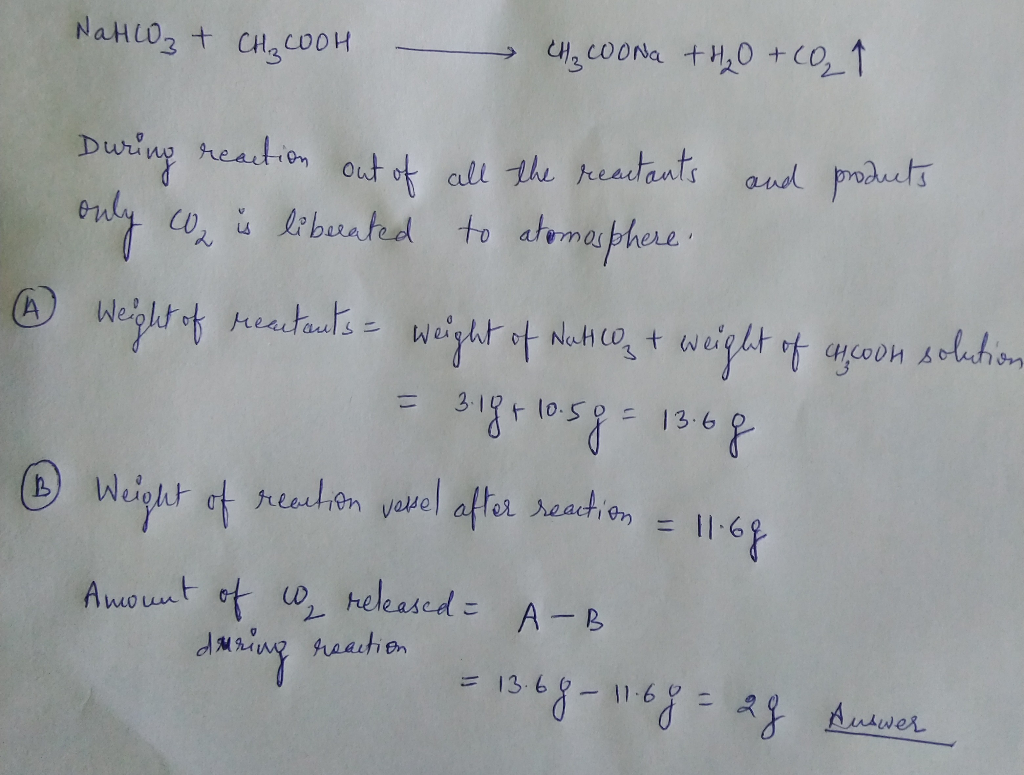#### Earn Coins

Coins can be redeemed for fabulous gifts.

Similar Homework Help Questions
• ### A 3.9 g sample of sodium hydrogen carbonate is added to a solution of acid weighing...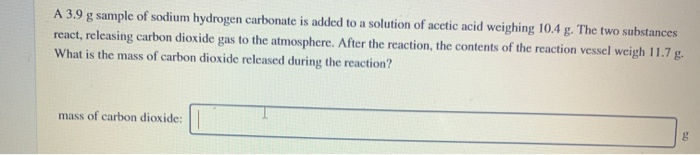A 3.9 g sample of sodium hydrogen carbonate is added to a solution of acid weighing 10.4 . The two substances react, releasing carbon dioxide gas to the atmosphere. After the reaction, the contents of the reaction vessel weigh What is the mass of carbon dioxide released during the reaction? A 3.9 g sample of sodium hydrogen carbonate is added to a solution of acetic acid weighing 10.4 g. The two substances react, releasing carbon dioxide gas to the atmosphere....

• ### 0 Assignment Score: 75.3% Resources Hint Check Answer Question 38 of 65 Chemistry: Fundamentals and Principles...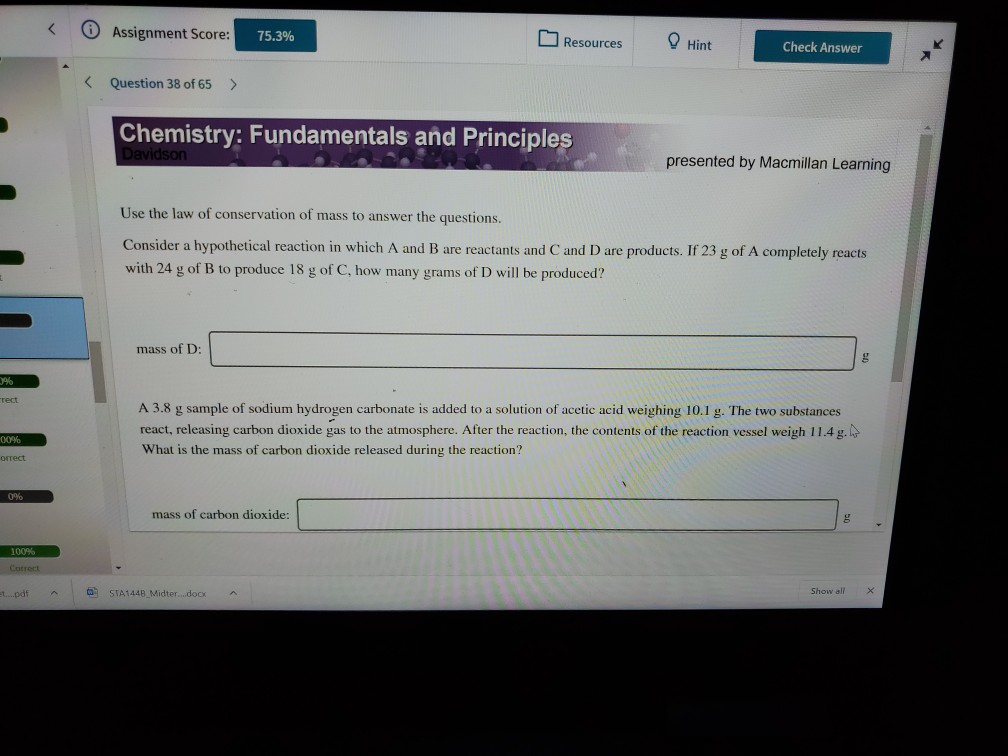0 Assignment Score: 75.3% Resources Hint Check Answer Question 38 of 65 Chemistry: Fundamentals and Principles Davidson presented by Macmillan Learning Use the law of conservation of mass to answer the questions. Consider a hypothetical reaction in which A and B are reactants and C and D are products. If 23 g of A completely reacts with 24 g of B to produce 18 g of C, how many grams of D will be produced? mass of D: 3% rect...

• ### Use the law of conservation of mass to answer the questions. Consider a hypothetical reaction in...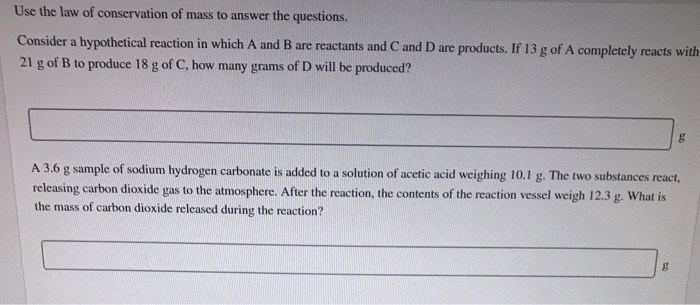Use the law of conservation of mass to answer the questions. Consider a hypothetical reaction in which A and B are reactants and C and D are products. If 13 g of A completely reacts with 21 g of B to produce 18 g of C, how many grams of D will be produced? A 3.6 g sample of sodium hydrogen carbonate is added to a solution of acetic acid weighing 10.1 g. The two substances react, releasing carbon dioxide...

• ### Sulfuric acid and sodium carbonate react to form carbon dioxide, water, and sodium sulfate as shown...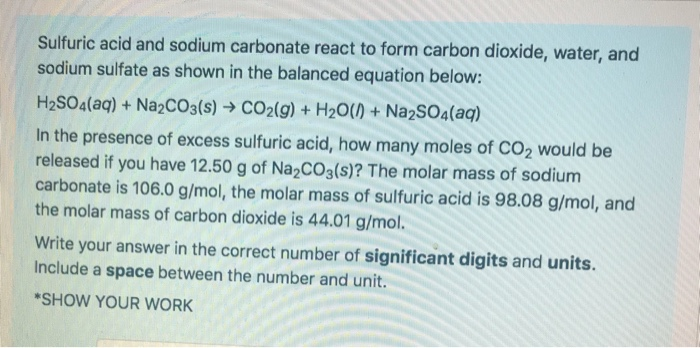Sulfuric acid and sodium carbonate react to form carbon dioxide, water, and sodium sulfate as shown in the balanced equation below: H2SO4(aq) + Na2CO3(s) + CO2(g) + H2O(1) + Na2SO4(aq) In the presence of excess sulfuric acid, how many moles of CO2 would be released if you have 12.50 g of Na2CO3(s)? The molar mass of sodium carbonate is 106.0 g/mol, the molar mass of sulfuric acid is 98.08 g/mol, and the molar mass of carbon dioxide is 44.01 g/mol....

• ### A 5.37 g mixture of sodium chloride and sodium carbonate is heated to decompose the sodium...

A 5.37 g mixture of sodium chloride and sodium carbonate is heated to decompose the sodium carbonate into sodium oxide and carbon dioxide according to the following chemical equation. Na2CO3 → Na2O + CO2 The sodium chloride will not decompose. If the final mixture has a mass of 4.03 g, what was the percent sodium carbonate in the original mixture? Hint: Consider the Law of Conservation of Matter - the mass of reactants must equal the mass of products. However,...

• ### 1.Sodium hydrogen carbonate, NaHCO3, can be decomposed completely by heating. 2 NaHCO3(s) → Na2CO3(s) + CO2(g)...

1.Sodium hydrogen carbonate, NaHCO3, can be decomposed completely by heating. 2 NaHCO3(s) → Na2CO3(s) + CO2(g) + H2O(g) A sample of impure NaHCO3 with an initial mass of 0.739 g yielded a solid residue (consisting of Na2CO3 and other solids) with a final mass of 0.564 g. Determine the mass percent of NaHCO3 in the sample. 2.In a process for producing acetic acid, oxygen gas is bubbled into acetaldehyde, CH3CHO, containing manganese(II) acetate (catalyst) under pressure at 60°C. 2CH3CHO(l) +...

• ### Extra Credit: (10 points) 15 a) A small amount of sodium hydrogen carbonate (NaHCO3) was added...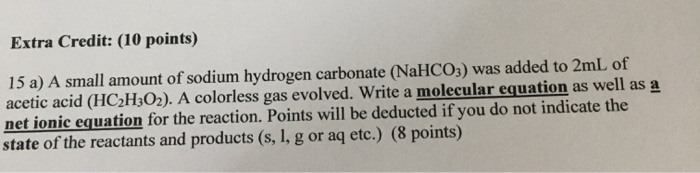Extra Credit: (10 points) 15 a) A small amount of sodium hydrogen carbonate (NaHCO3) was added to 2mL of acetic acid (HC2H302). A colorless gas evolved. Write a molecular equation as well as a net ionic equation for the reaction. Points will be deducted if you do not indicate the state of the reactants and products (s, 1, g or aq etc.) (8 points)

• ### A sample of sodium reacts completely with 0.497 kg of chlorine, forming 819 g of sodium...

A sample of sodium reacts completely with 0.497 kg of chlorine, forming 819 g of sodium chloride. What mass of sodium reacted? Express your answer to three significant figures and include the appropriate units How many moles of methane are produced when 48.1 moles of carbon dioxide gas react with excess hydrogen gas? Express your answer with the appropriate units. How many moles of hydrogen gas would be needed to react with excess carbon dioxide to produce 76.6 moles of...

• ### An antacid tablet weighing 0.943g contained calcium carbonate as the active ingredient, in addition to an...

An antacid tablet weighing 0.943g contained calcium carbonate as the active ingredient, in addition to an inert binder. When an acid solution weighing 56.398 g was added to the tablet, carbon dioxide gas was released, producing a fizz. The resulting solution weighed 57.136 g. How many grams of carbon dioxide were produced?

• ### A 18.5 g sample of a mixture of magnesium carbonate and calcium carbonate is treated with...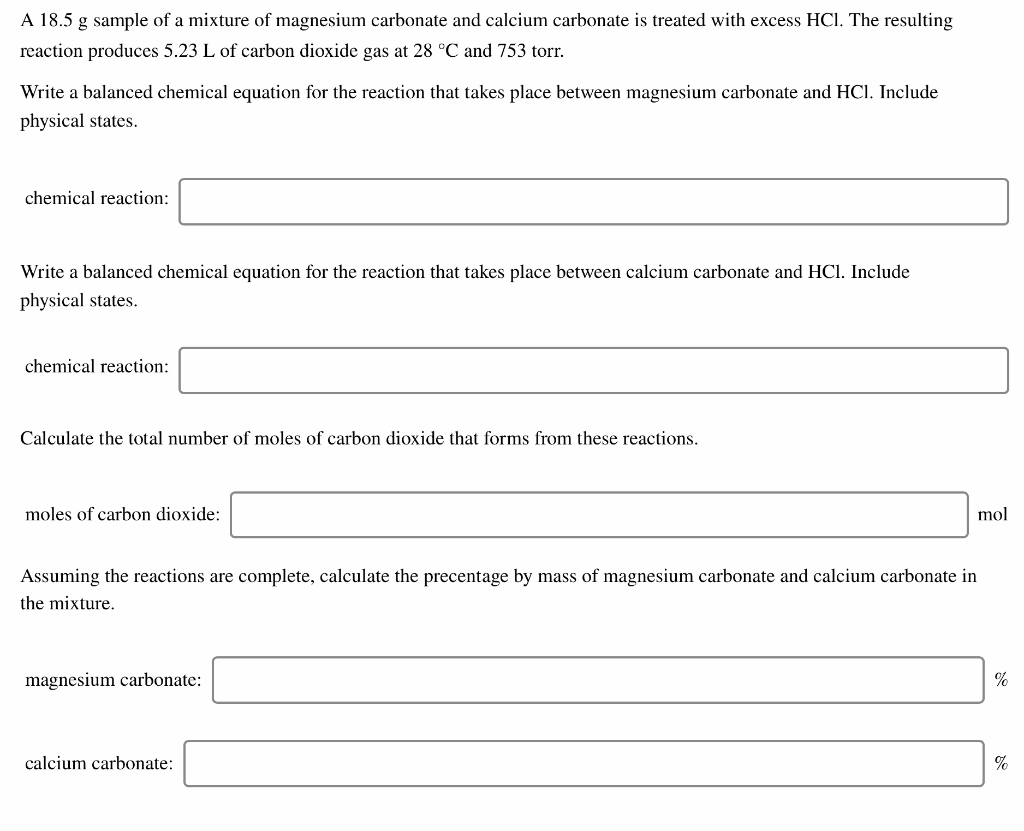A 18.5 g sample of a mixture of magnesium carbonate and calcium carbonate is treated with excess HCl. The resulting reaction produces 5.23 L of carbon dioxide gas at 28 °C and 753 torr. Write a balanced chemical equation for the reaction that takes place between magnesium carbonate and HCl. Include physical states. chemical reaction: Write a balanced chemical equation for the reaction that takes place between calcium carbonate and HCl. Include physical states. chemical reaction: Calculate the total number...